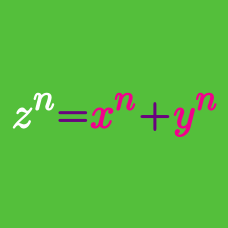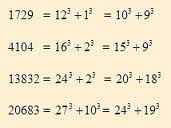Number Theory

# General Diophantine Equations: Level 3 Challenges1729 can be expressed as the sum of two perfect cubes in two distinct ways.

How many such numbers exist?

Hint: Given one set of such numbers, how could you construct another set?

If $a$ and $b$ are integers such that $a^3+b^3=2015$, then what is $a+b$?

$\LARGE{2 + 2 = 2 \times 2 \\ 1 + 2 + 3 = 1 \times 2 \times 3}$

In the above equations, there are respectively 2 and 3 positive integers whose sum is equal to their product.

Find 4 positive integers whose sum is equal to their product. Enter the answer as their sum

Find the positive integer $n$ such that $n^3 + 2n^2 + 9n + 8$ is a perfect cube.

$a + b + c + d + e = a \times b \times c \times d \times e$

How many unordered 5-tuples of positive integers are there which satisfy the above equation?

×